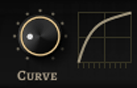# Knob for adjusting the velocity curve on the Macro Page

Hi
I need to insert a couple of knobs in a Macro Page, knobs for adjusting the velocity curve of certain layers. Something like Halion’s Eagle and Raven have:Both Eagle and Raven have a script that accomplish this, and I tried taking some parts of that script and adapt it, but as that script is pretty intricate, with plenty of functions and parameters (a lot of them for other elements of Eagle/Raven’s MacroPage) I couldn’t figure out how to make it work in my case.

The graph showing the curve is not extremely important in my case, the knob would probably be enough. But if it’s not very complicated to implement, then having that graph as well would be nice.

Any ideas?

You could try something like this:

``````-- parameters for volume bending
defineParameter("curve", "Dynamics Curve", 0, -1, 1, 0, function() onCurveChanged() end)
defineParameter("lowerLimit", "Minimum Level", 0, 0, 127, 1, function() onLowerLimitChanged() end)
defineParameter("upperLimit", "Minimum Level", 127, 0, 127, 1, function() onUpperLimitChanged() end)
-- envelope parameter for curve display
defineParameter{name="curvePoints", default={{level = 0, curve = 0, duration = 0}, {level = 1, curve = 0, duration = 1}}, type="Envelope"}

function onCurveChanged()
curvePoints.curve = curve
end

function onLowerLimitChanged()
curvePoints.level = lowerLimit / 127
end

function onUpperLimitChanged()
curvePoints.level = upperLimit / 127
end

function scaleVelocity(velocity)
local volume = velocity / 127
local volume2
if curve > 0 then
volume = math.pow(volume, 1 + 2 * curve)
elseif curve < 0 then
volume = 1 - math.pow(1 - volume, 1 + 2 * math.abs(curve))
end
volume = (upperLimit / 127 - lowerLimit / 127) * volume + lowerLimit / 127
volume = math.floor(volume * 127)
print(velocity, volume)
return volume
end

function onNote(e)
e.velocity = scaleVelocity(e.velocity)
postEvent(e)
end
``````

Velocity Curve.vstpreset (13.4 KB)

Or you could use the Velocity Curve midi module and the Curve Editor template.

1 Like

Thank you so much @misohoza ! You’re very helpful as always.I implemented your script and it works perfectly!But there are two things in the script that are not clear to me. First one:

function scaleVelocity(velocity)
local volume = velocity / 127
local volume2

What’s the role of that “local volume2”? Cause it doesn’t appear anywhere else in the script

And the other one:

volume = (upperLimit / 127 - lowerLimit / 127) * volume + lowerLimit / 127
volume = math.floor(volume * 127)

I understand that the “volume” is the resulting velocity (after the curve is applied) but why is it calculated in two ways (one time as (upperLimit / 127 - lowerLimit / 127) * volume + lowerLimit / 127 and then as math.floor(volume * 127) ) ?

My mistake. I tried another way of calculation and then wanted to compare the results. Just forgot to delete that line. So simply delete that. It doesn’t do anything right now.

When you do the calculations you can end up with decimal number. So the math floor is there to round it down to integer. You could combine the two lines into one or just ignore the math floor. It should work too.

1 Like

Ah, I see! It all makes sense now. Thanks a lot for the explanation!